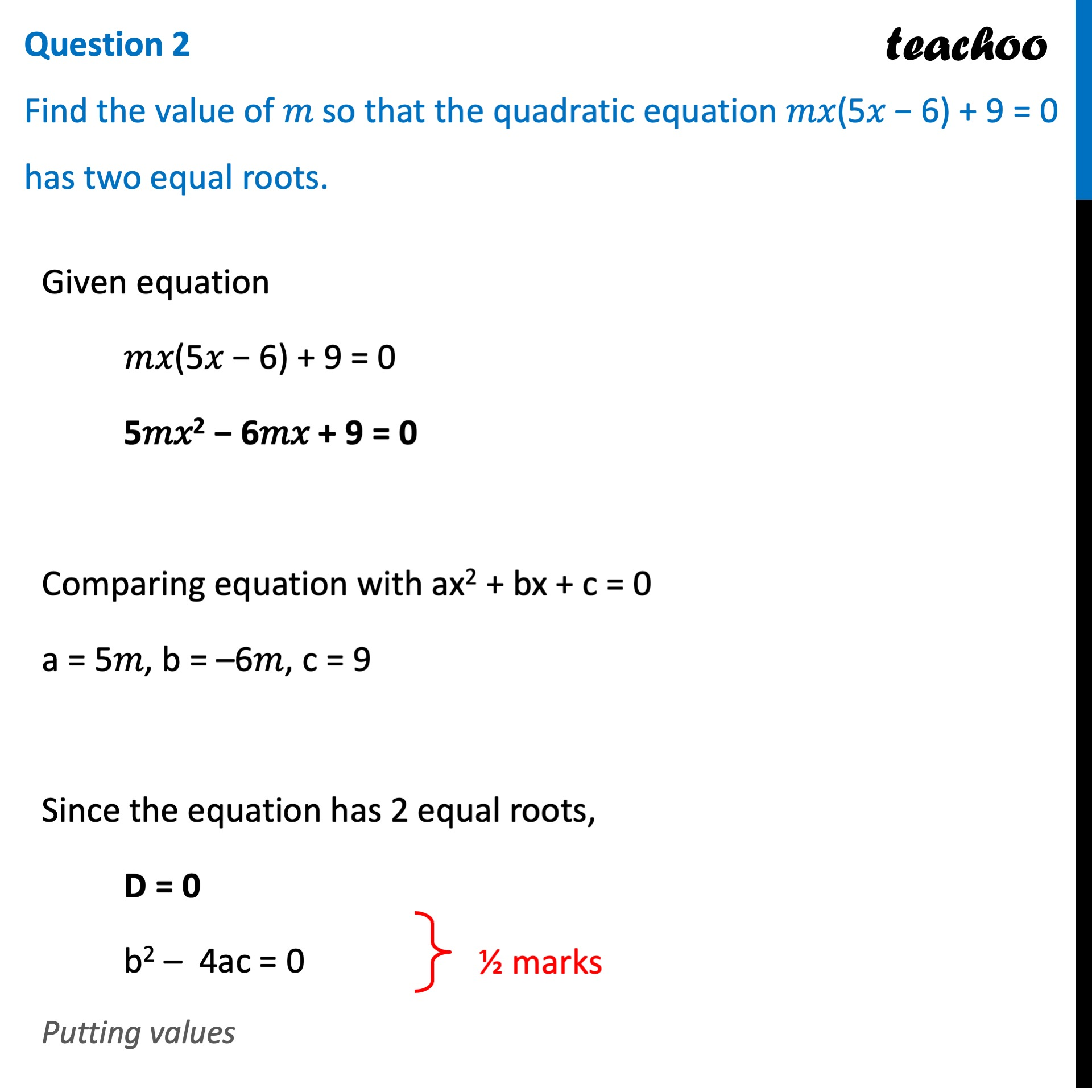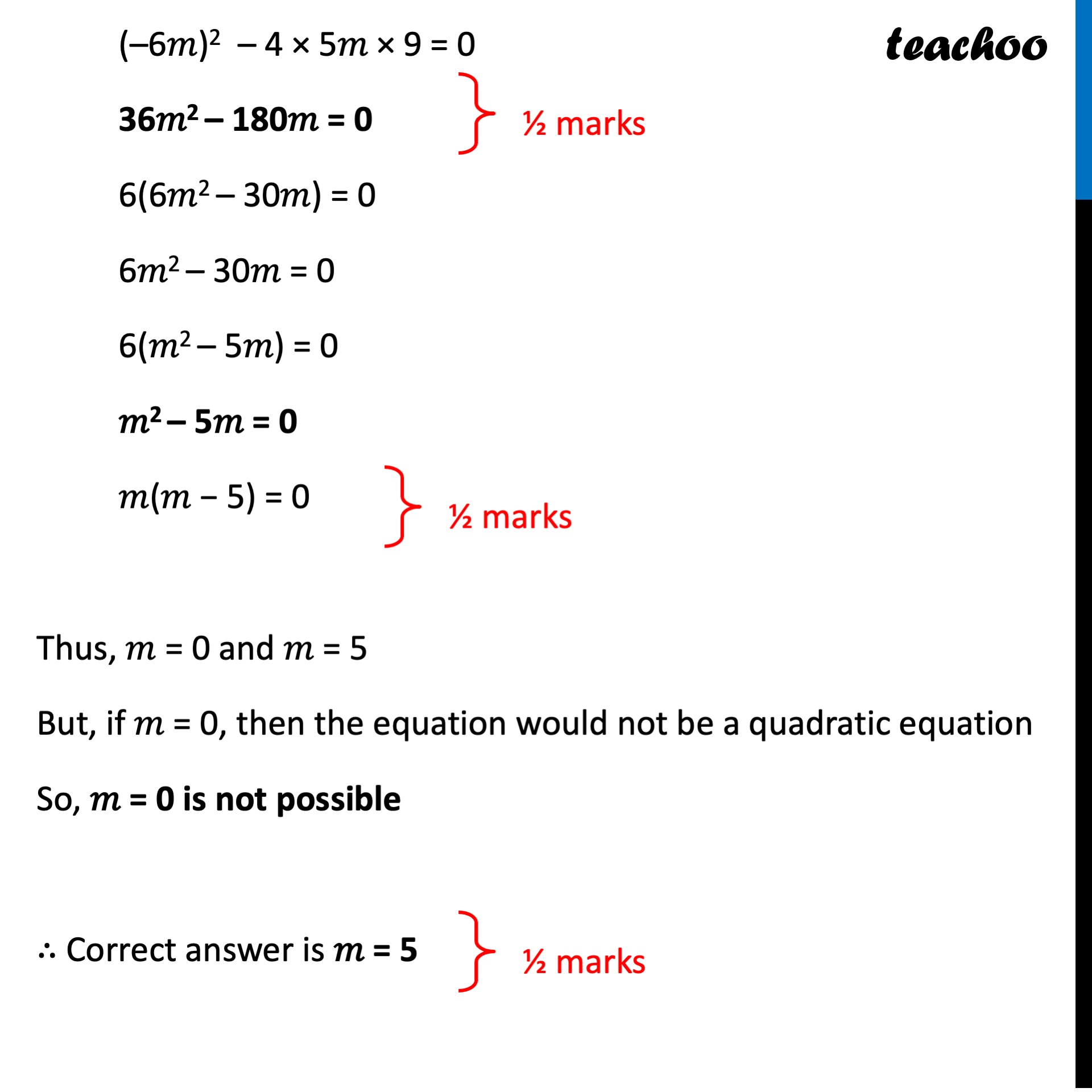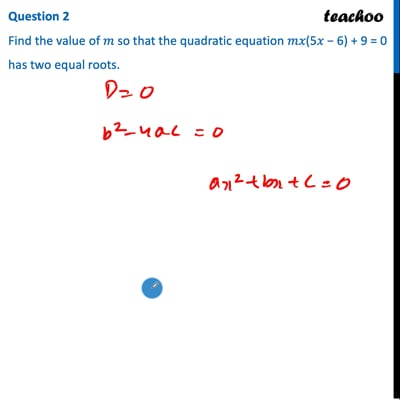CBSE Class 10 Sample Paper for 2022 Boards - Maths Standard [Term 2]

Class 10
Solutions of Sample Papers for Class 10 Boards

## Find the value of m so that the quadratic equation mx (5x - 6) + 9 = 0 has two equal rootsThis video is only available for Teachoo black users

Learn in your speed, with individual attention - Teachoo Maths 1-on-1 Class

### Transcript

Question 2 Find the value of 𝑚 so that the quadratic equation 𝑚𝑥(5𝑥 − 6) + 9 = 0 has two equal roots. Given equation 𝑚𝑥(5𝑥 − 6) + 9 = 0 5𝑚𝑥2 − 6𝑚𝑥 + 9 = 0 Comparing equation with ax2 + bx + c = 0 a = 5𝑚, b = –6𝑚, c = 9 Since the equation has 2 equal roots, D = 0 b2 – 4ac = 0 Putting values (–6𝑚)2 – 4 × 5𝑚 × 9 = 0 36𝑚2 – 180𝑚 = 0 6(6𝑚2 – 30𝑚) = 0 6𝑚2 – 30𝑚 = 0 6(𝑚2 – 5𝑚) = 0 𝑚2 – 5𝑚 = 0 𝑚(𝑚 − 5) = 0 Thus, 𝑚 = 0 and 𝑚 = 5 But, if 𝑚 = 0, then the equation would not be a quadratic equation So, 𝑚 = 0 is not possible ∴ Correct answer is 𝑚 = 5